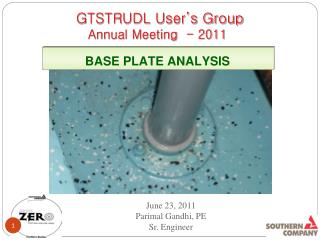Download PresentationGTSTRUDL User’s Group

# GTSTRUDL User’s Group

Download Presentation## GTSTRUDL User’s Group

- - - - - - - - - - - - - - - - - - - - - - - - - - - E N D - - - - - - - - - - - - - - - - - - - - - - - - - - -
##### Presentation Transcript

1. GTSTRUDL User’s Group Annual Meeting - 2011 BASE PLATE ANALYSIS June 23, 2011Parimal Gandhi, PE Sr. Engineer

2. PURPOSE • Non-Linear Finite Element Analysis For Base Plate. • AND • Stiffness Analysis For Base Plate.

3. AGENDA • INTRODUCTION AND THEORY • BASE PLATE MODELING • EDIT THE BASE PLATE INPUT FILE • BASE PLATE STRESSES & BOLTS LOADS • FLEXIBLE & RIGID BASE PLATE RESULTS • CONCLUSION

4. Introduction & Theory • The finite element method is used to analyze the base plate considering the beam on elastic foundation. • The concrete and bolts are represented by non linear spring elements attached to the nodal points on the steel base plate and to the ground. • The steel base plate is modeled with SBHQ6 • and SBHT6 plate bending element. • Attachments and Stiffeners are modeled as RIGID SOLIDS in the plane of the base plate.

5. Introduction & Theory The Beam on Elastic Foundation

6. Introduction & Theory • Non Linear Spring Element • Concrete

7. Introduction & Theory • Non Linear Spring Element • Bolt

8. Introduction & Theory Non-linear Spring Curves

9. Base Plate Modeling • Base Plate Data • Base Plate: ¾” x 12” Dia • 4 ( Four) 5/8” Hilti Kwik Bolt 3 • @ 5” Radius With Minimum • Embed 4” • Attachment : 4” Sch. 40 Pipe • Concrete : f’c = 4000 psi

10. Base Plate Modeling

11. Circular Base Plate Modeling

12. Circular Base Plate Modeling

13. Circular Base Plate Modeling

14. Circular Base Plate Modeling

15. Circular Base Plate Modeling

16. Circular Base Plate Modeling

17. Circular Base Plate Modeling

18. Circular Base Plate Modeling Elements=24x12=288 Joints=289 Joint 289 Element 288

19. Edit Base Plate Input File The GT Model Wizard creates a GTSTRUDL input file that remains after the Wizard is closed.

20. Edit Base Plate Input File Soil Spring Values

21. Edit Base Plate Input File For Attachment Master Joint ‘C1’ Slave Joints 74 to 97

22. Edit Base Plate Input File For Concrete Stiffness

23. Edit Base Plate Input File For Concrete Stiffness

24. Edit Base Plate Input File For Bolt Stiffness

25. Edit Base Plate Input File For Bolt Stiffness

27. Base Plate Stresses

28. Bolt Support Reactions

29. Square /Rectangular Base Plate Modeling • Base Plate Data • Base Plate: ¾” x 12” x 12” • 4 ( Four) 5/8” Hilti Kwik Bolt 3 • @ 10” c/c With Minimum • Embed 4” • Attachment : TS 4x4x3/8 • Concrete : f’c = 4000 psi

30. Square /Rectangular Base Plate Modeling

31. Square /Rectangular Base Plate Modeling

32. Square /Rectangular Base Plate Modeling

33. Base Plate odeling Square /Rectangular Base Plate Modeling

34. Square /Rectangular Base Plate Modeling

35. Square /Rectangular Base Plate Modeling

36. Square /Rectangular Base Plate Modeling

37. Base Plate Modeling Square /Rectangular Base Plate Modeling

38. Square /Rectangular Base Plate Modeling

39. Square /Rectangular Base Plate Modeling

40. Square /Rectangular Base Plate Modeling

41. Square /Rectangular Base Plate Modeling

42. Square /Rectangular Base Plate Modeling X Total Joints =169 Total Elements =144 z

43. Square /Rectangular Base Plate Modeling Master Joint ‘C1’ Spring Element ‘S1’ Spring Element ‘S169’ Slave Joints 57, 61 … 113

44. Edit Base Plate Input File LOADING Non Linear Analysis `` Output

45. Square /Rectangular Base Plate Stresses B3 B4 B1 B2

46. Support Reactions for Bolts

47. Edit Rigid Base Plate Input File Rigid Attachment Bolt : Pinned Support `` Loading `` Stiffness Analysis `` Output

48. Flexible & Rigid Base Plate Result Flexible Rigid 339 lbs 423 lbs 949 lbs 949 lbs 9215 psi 9404 psi Δy=0.00361” Δy=0.00432”

49. THE END Questions? Thank you.